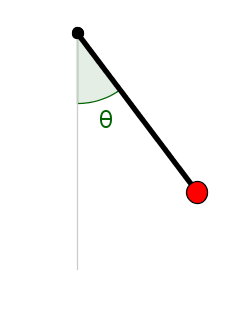# Math Insight

### Image: Pendulum positionWe can represent the position of a pendulum by the angle $\theta$ it makes with straight down. The angle $\theta=0$ corresponds to the pendulum pointing straight down, and $\theta=\pm\pi$ corresponds to the pendulum pointing straight up. We use the convention that $0 < \theta< \pi$ indicates that the pendulum is pointing toward the right and that $-\pi < \theta< 0$ indicates that the pendulum is pointing toward the left.

Image file: pendulum_position.png

Source image file: pendulum_position.ggb
Source image type: Geogebra